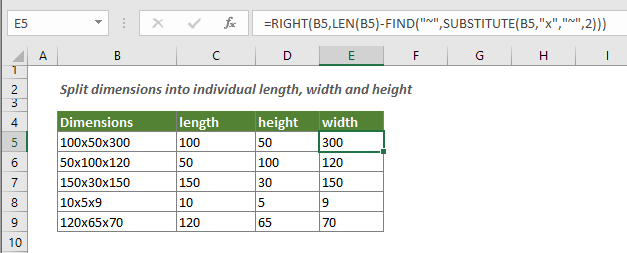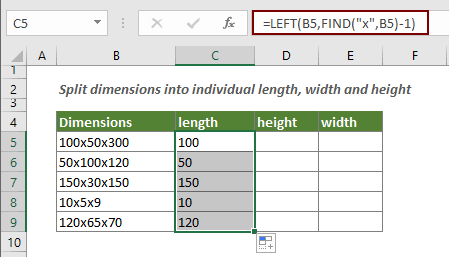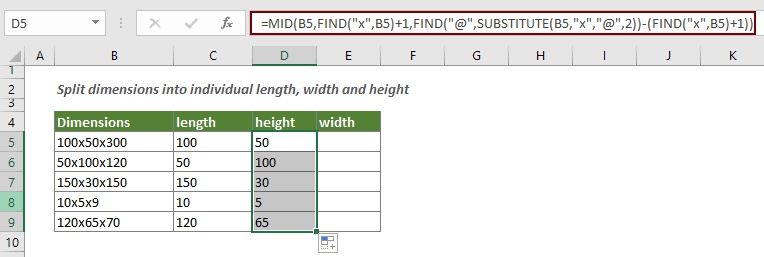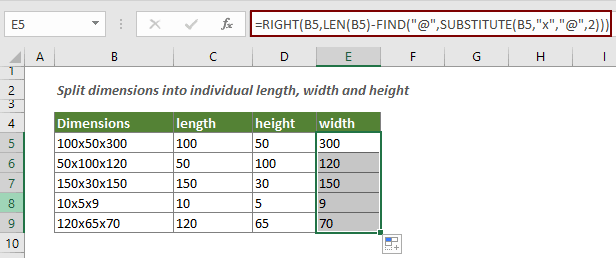## Split dimensions into individual length, height and width

This article explains how to split dimensions in a cell into three parts (individual dimensions that include length, height and width).#### How to split dimensions into three parts?

To split dimensions into individual length, height and width, you need to apply three different formulas separately.

Generic formula of extracting the first dimension

=LEFT(text,FIND("char",text)-1)

Generic formula of extracting the second dimension

=MID(text,FIND("char",text)+1,FIND("@",SUBSTITUTE(text,"char","@",2))-(FIND("char",text)+1))

Generic formula of extracting the third dimension

=RIGHT(text,LEN(text)-FIND("@",SUBSTITUTE(text,"char","@",2)))

Arguments of above formulas

Text: The string you want to extract the given number of characters from it. It can be:

• 1. The text enclosed in quotation marks;
• 2. Or a reference to a cell containing the text.

Char: The character you will split the dimensions based on.

How to use these formulas?

##### Firstly, we need to get the first dimension.

1. Select a blank cell to output the first dimension.

2. Enter the below formula into it and press the Enter key. Selecting the result cell and drag the Fill Handle to apply the formula to other cells as you need.

=LEFT(B5,FIND("x",B5)-1)##### Then, we are going to splitting the second dimension as follows.

1. Select a blank cell to output the second dimension.

2. Enter the below formula into it and press the Enter key. Selecting the result cell and drag the Fill Handle to apply the formula to other cells as you need.

=MID(B5,FIND("x",B5)+1,FIND("@",SUBSTITUTE(B5,"x","@",2))-(FIND("x",B5)+1))##### Finally, get the last dimension

1. Select a blank cell to output the last dimension.

2. Enter the below formula into it and press the Enter key. Selecting the result cell and drag the Fill Handle to apply the formula to other cells as you need.

=RIGHT(B5,LEN(B5)-FIND("@",SUBSTITUTE(B5,"x","@",2)))How these formulas work?

=LEFT(B5,FIND("x",B5)-1)

1. FIND("x",B5)-1: The FIND function gets the position of the first "x" in B5. For getting the first dimension without the first "x", you need to subtract 1. Then the result is 4-1=3.
2. LEFT(B5,3): The LEFT function extract 3 characters starting from the left side of B5 and returns the result as 100.

=MID(B5,FIND("x",B5)+1,FIND("@",SUBSTITUTE(B5,"x","@",2))-(FIND("x",B5)+1))

1. FIND("x",B5)+1: The FIND function locates the first "x" of B5. As you need to get the second dimension after the first "x", here adding 1 is needed. Then the result is 4+1=5.
2. FIND("@",SUBSTITUTE(B5,"x","@",2)):

• SUBSTITUTE(B5,"x","@",2): The SUBSTITUTE function replaces the second "x" in B5 with "@" and returns the result as 100x50@300;
• FIND("@",100x50@300): The FIND function here gets the position of the "@" character in the text 100x50@300, and returns the result as 7.

3. (FIND("x",B5)+1): As above step 1 mentioned, here the FIND function locates the first "x" of B5 and adds 1 to return the result as 5.
4. MID(B5,5,7-(5)): The MID function extracts two characters (7-5=2) starting at the fifth character of the text in B5. And return the final result as 50.

=RIGHT(B5,LEN(B5)-FIND("@",SUBSTITUTE(B5,"x","@",2)))

1. LEN(B5): The LEN function calculates the total string length in B5 and returns the result as 10;
2. FIND("@",SUBSTITUTE(B5,"x","@",2)):

• SUBSTITUTE(B5,"x","@",2): The SUBSTITUTE function replaces the second "x" in B5 with "@" and returns the result as 100x50@300;
• FIND("@",100x50@300): The FIND function here locates the "@" in the text 100x50@300, and returns the result as 7.

3. LEN(B5)-FIND("@",SUBSTITUTE(B5,"x","@",2)): (10-7) The total length of the text string in cell B5 subtracts the first 7 characters, and returns the result as 3.
4. RIGHT(B5,3): And then the RIGHT function extracts 3 characters starting from the right side of B5 and returns the third dimension as 300.

#### Related functions

Excel LEFT function
The LEFT function extracts the given number of characters from the left side of a supplied string.

Excel MID function
MID function is used to find and return a specific number of characters from the middle of given text string.

Excel RIGHT function
The RIGHT function extracts a specific number of characters from the right side of the text string.

Excel FIND function
The FIND function is used to find a string within another string, and returns the starting position of the string inside another one.

Excel LEN function
The LEN function returns the number of characters in a text string.

Excel SUBSTITUTE function
The Microsoft Excel SUBSTITUTE function replaces text or characters within a text string with another text or characters.

#### Related formulas

Split dimensions into two parts in Excel
This article explains how to use formula to split dimensions in a cell into two parts without units (individual length and width).

Separate numbers from units of measurement
This article explains how to use formula to separate numbers from unites of measurement.

### The Best Office Productivity Tools

#### Kutools for Excel - Helps You To Stand Out From Crowd

Would you like to complete your daily work quickly and perfectly? Kutools for Excel brings 300 powerful advanced features (Combine workbooks, sum by color, split cell contents, convert date, and so on...) and save 80% time for you.

• Designed for 1500 work scenarios, helps you solve 80% Excel problems.
• Reduce thousands of keyboard and mouse clicks every day, relieve your tired eyes and hands.
• Become an Excel expert in 3 minutes. No longer need to remember any painful formulas and VBA codes.
• 30-day unlimited free trial. 60-day money back guarantee. Free upgrade and support for 2 years.#### Office Tab - Enable Tabbed Reading and Editing in Microsoft Office (include Excel)

• One second to switch between dozens of open documents!
• Reduce hundreds of mouse clicks for you every day, say goodbye to mouse hand.
• Increases your productivity by 50% when viewing and editing multiple documents.
• Brings Efficient Tabs to Office (include Excel), Just Like Chrome, Firefox, And New Internet Explorer.